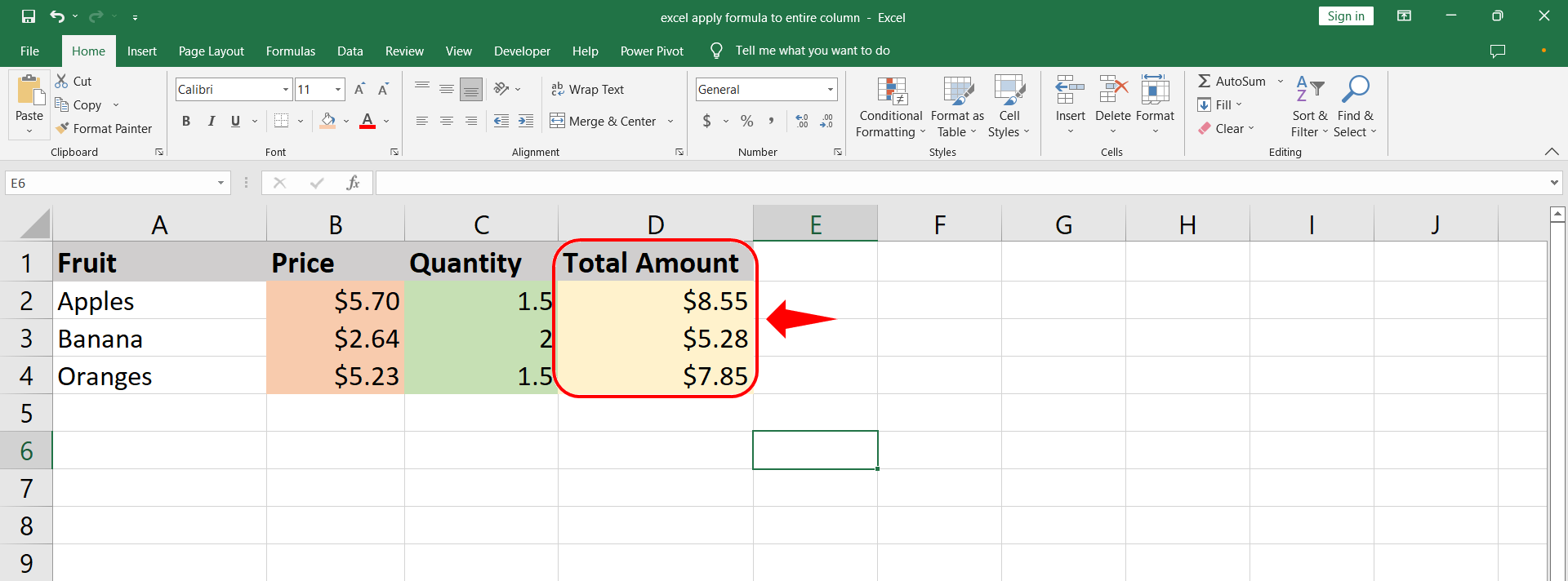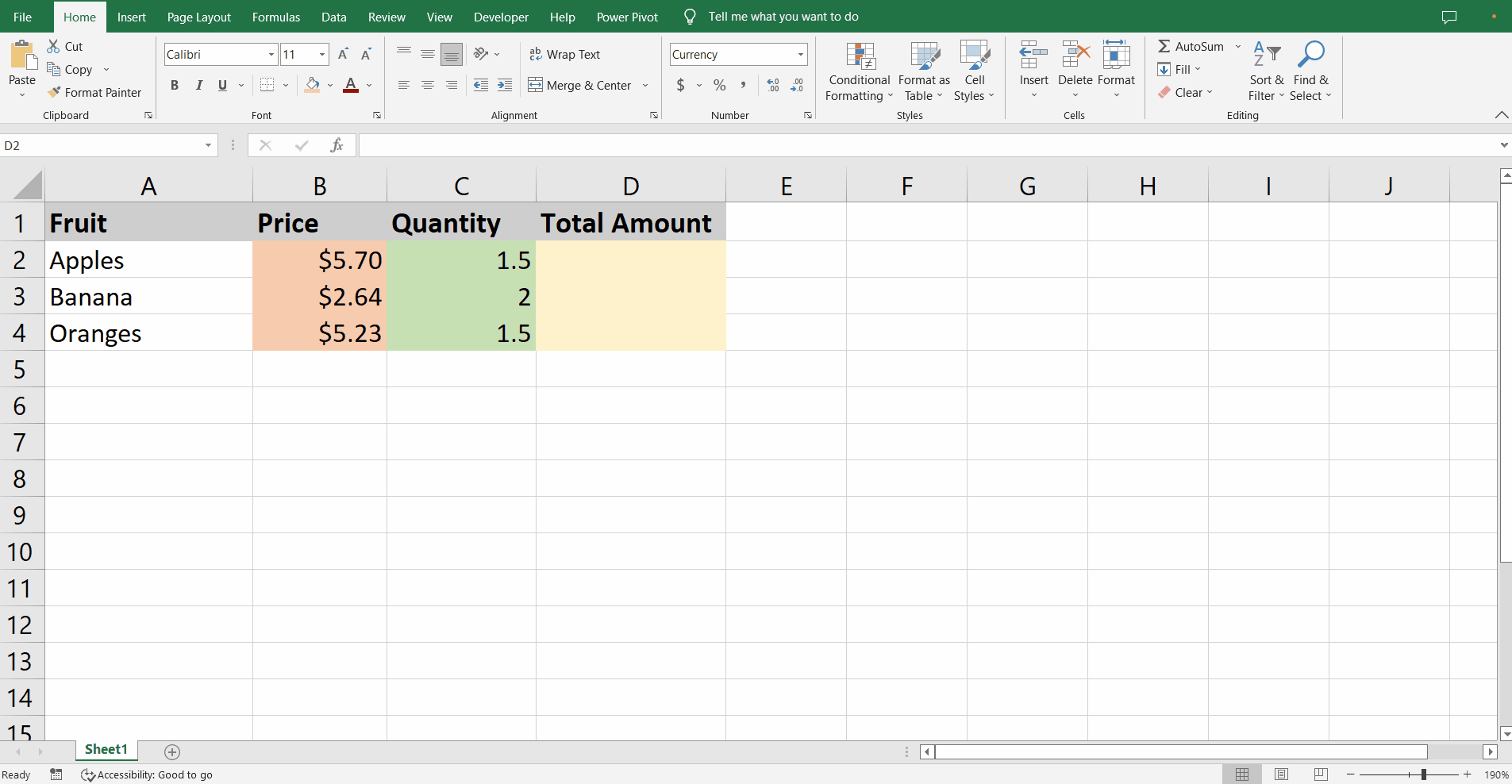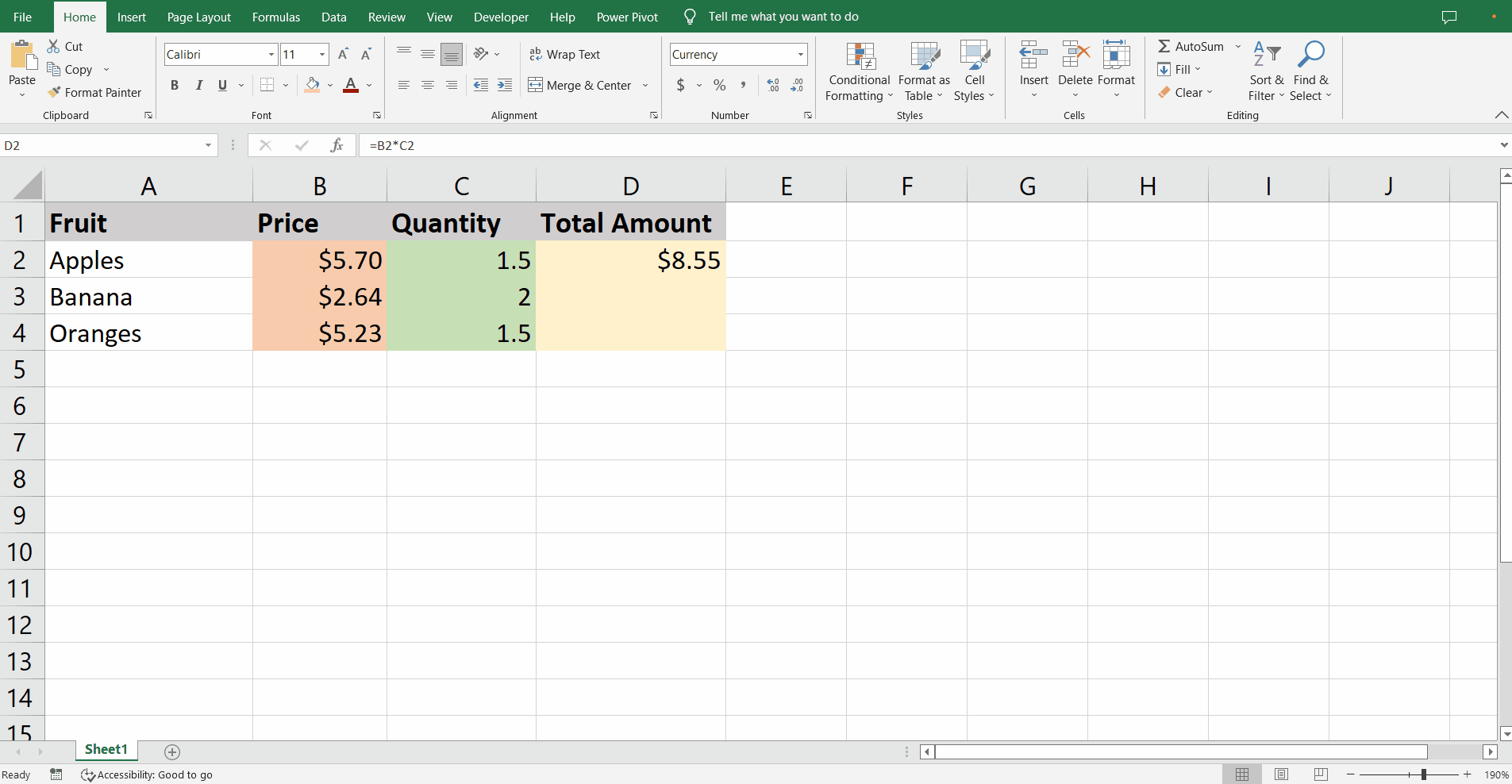# How to apply formula to entire column in Excel

We have a dataset of fruit prices and quantity and we need to calculate the total cost of each fruit type. The dataset is shown in the picture above.One of the strongest features of excel is the relative referencing. This feature helps us to apply formulas to the entire column swiftly without errors. So, let’s dive into it.

### Step 1 – Implement formula– In the cell D2 start typing the formula with equal sign followed by selection of cell B2.

– Then type the asterisk * and click cell C2.

– Press the key combination Ctrl + Enter and the formula is loaded into the cell.

– The Ctrl+Enter combination keeps the same cell D2 selected.

– The result of multiplication of values in cells B2 and C2 is visible in cell D2.

### Step 2 – Use Fill Handle– The bottom right corner of a selected cell has a green cross known as a Fill Handle.

– Drag the Fill Handle to the last row to apply the formula to the entire column.

– If the dataset is huge you can double click to apply the formula till the empty row is encountered.